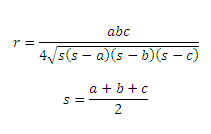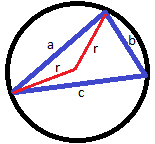# Circle circumscribing Triangle Calculator

This CalcTown calculator calculates the circumscribing circle radius, its area and perimeter.

#### Result

m
m2
mClick here to view image

Where,

π = 3.14 (constant)

s = semi-perimeter of the circumscribing circle

r = radius of the circumscribing circle